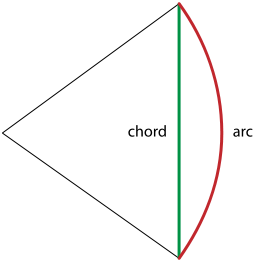A# chordChord of an arc.

A chord is a straight line that joins two points on a curve. The chord of an arc is a line joining its two extremities. Most commonly, chord is used to mean a straight line segment joining, and included between, two points on a circle, ellipse, parabola, etc. In the restricted sense of a chord of circle the term first appears in English in 1551 in Robert Recorde's The Pathwaie to Knowledge: "Defin., If the line goe crosse the circle, and passe beside the centre, then is it called a corde, or a stryngline."

If we draw a series of parallel chords in any conic, the line through their middle points is called a diameter, and a line parallel to the chords which passes through the extremity of the diameter is a tangent to the curve. Hence in the circle (1) a diameter is perpendicular to the chords which it bisects, and also (2) to the tangent at its extremity; (3) the three chords of intersection of any three circles meet in a point. In any conic section the tangents at the ends of any chord meet in the diameter which bisects the chord.

Some surprising results emerge from moving chords. For example, take a chord in a circle C, and slide the chord around the circle so that the midpoint of the chord traces out a smaller concentric circle. Call the area between the two circles A(C). Now do the same thing with a larger circle C' but with the same length chord. Is A(C') larger or smaller than A(C)? Surprisingly, they are the same. In other words A(C) doesn't depend on what circle we start with, only the length of the chord. An even more amazing fact is that if we slide a chord of fixed length around any convex shape C so that the chord's midpoint traces out another figure D, the area between C and D doesn't depend on what shape we started with.